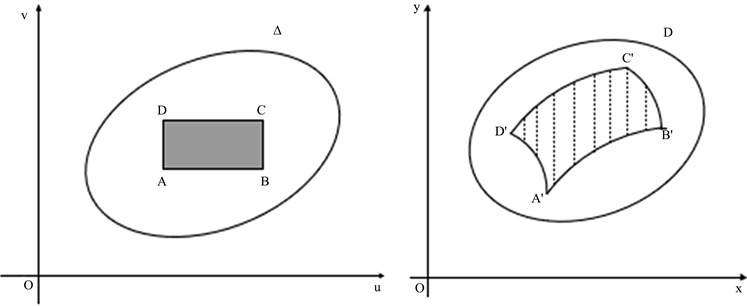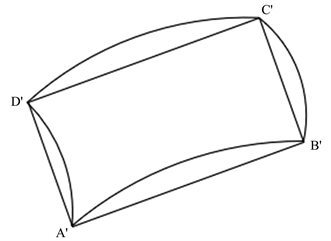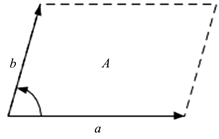# 体积元几何与Jacobi行列式之关系The Relationship between Volume Element Geometry Related and Jacobi Determinant

DOI: 10.12677/AAM.2021.104137, PDF, HTML, XML, 下载: 9  浏览: 29

Abstract: In the study of mathematical analysis, we have learned the solving methods of arc length, area and volume, and how to use these methods to find physical quantities such as the mass of an object and the moment of inertia, which is a very important part in our learning process. In order to ensure that we have a better understanding of the content in the learning process, this thesis will introduce the relationship between volume elements and Jacobi rows from three different aspects of coordinate transformation, vector exterior product and vector inner product.

1. 引言

2. 弧长、面积、体积元的表示与Jacobi行列式

2.1. 平面曲线的弧长

$x=x\left(t\right),\text{}y=y\left(t\right),\text{}t\in \left[\alpha ,\beta \right]$ (1)Figure 1. Differential triangle

$\text{d}s=\sqrt{\text{d}{x}^{2}+\text{d}{y}^{2}}=\text{\hspace{0.17em}}\sqrt{{{x}^{\prime }}^{2}\left(t\right)+{{y}^{\prime }}^{2}\left(t\right)}\text{d}t$ (2)

$r=r\left(\theta \right),\text{}\theta \in \left[\alpha ,\beta \right]$ (3)

$x=r\left(\theta \right)\mathrm{cos}\theta ,\text{}y=r\left(\theta \right)\mathrm{sin}\theta ,\text{}\theta \in \left[\alpha ,\beta \right]$

${x}^{\prime }\left(\theta \right)={r}^{\prime }\left(\theta \right)\mathrm{cos}\theta -r\left(\theta \right)\mathrm{sin}\theta ,$

${y}^{\prime }\left(\theta \right)={r}^{\prime }\left(\theta \right)\mathrm{sin}\theta +r\left(\theta \right)\mathrm{cos}\theta ,$

${{x}^{\prime }}^{2}\left(\theta \right)+{{y}^{\prime }}^{2}\left(\theta \right)={r}^{2}\left(\theta \right)+{{r}^{\prime }}^{2}\left(\theta \right),$

$\text{d}s=\sqrt{{r}^{2}\left(\theta \right)+{{r}^{\prime }}^{2}\left(\theta \right)}\text{d}\theta .$

$y=f\left(x\right),\text{}x\in \left[a,b\right]$ (4)

$x=x,\text{}y=f\left(x\right),\text{}x\in \left[a,b\right].$

$\text{d}s=\sqrt{1+{{f}^{\prime }}^{2}\left(x\right)}\text{d}x.$

$s={\int }_{\alpha }^{\beta }\sqrt{{{x}^{\prime }}^{2}\left(t\right)+{{y}^{\prime }}^{2}\left(t\right)}\text{d}t.$

$s={\int }_{\alpha }^{\beta }\sqrt{{r}^{2}\left(\theta \right)+{{r}^{\prime }}^{2}\left(\theta \right)}\text{\hspace{0.17em}}\text{d}\theta .$

$s={\int }_{\alpha }^{\beta }\sqrt{1+{{f}^{\prime }}^{2}\left(x\right)}\text{\hspace{0.17em}}\text{d}x.$

2.2. 第一型曲线积分

$L:\left\{\begin{array}{c}x=\phi \left(t\right),\\ y=\psi \left(t\right),\end{array}\text{}t\in \left[\alpha ,\beta \right],$

${\int }_{L}f\left(x,y\right)\text{d}s={\int }_{\alpha }^{\beta }f\left(\phi \left(t\right),\psi \left(t\right)\right)\sqrt{{{\phi }^{\prime }}^{2}\left(t\right)+{{\psi }^{\prime }}^{2}\left(t\right)}\text{d}t.$ (5)

$y=\psi \left(x\right),\text{}x\in \left[a,b\right]$

$\underset{L}{\int }f\left(x,y\right)\text{d}s={\int }_{a}^{b}f\left(x,\psi \left(x\right)\right)\sqrt{{{\phi }^{\prime }}^{2}\left(x\right)+{{\psi }^{\prime }}^{2}\left(x\right)}\text{d}x.$

$\text{d}s=\text{\hspace{0.17em}}\sqrt{{{\phi }^{\prime }}^{2}\left(t\right)+{\psi }^{2}\left(t\right)+{{\chi }^{\prime }}^{2}\left(t\right)}\text{d}t$

$\underset{L}{\int }f\left(x,y,z\right)\text{d}s={\int }_{\alpha }^{\beta }f\left(\phi \left(t\right),\psi \left(t\right),\chi \left(t\right)\right)\sqrt{{{\phi }^{\prime }}^{2}\left(t\right)+{{\psi }^{\prime }}^{2}\left(t\right)+{{\chi }^{\prime }}^{2}\left(t\right)}\text{d}t.$

2.3. 二重积分的变量变换与面积微元

$J\left(u,v\right)=\frac{\partial \left(x,y\right)}{\partial \left(u,v\right)}\ne 0,\text{}\left(u,v\right)\in \text{Δ,}$

$\text{Δ}$$xy$ 平面上的对应区域D之间的微元面积伸缩系数为 $|J\left(u,v\right)|$，即

$\text{d}x\text{d}y=|J\left(u,v\right)|\text{d}u\text{d}v.$Figure 2. Area micro-element transformation

${A}^{\prime }:{x}_{1}=x\left(u,v\right),\text{}{y}_{1}=y\left(u,v\right);$

$\begin{array}{l}{B}^{\prime }:{x}_{2}=x\left(u+\text{d}u,v\right)=x\left(u,v\right)+\frac{\partial x\left(u,v\right)}{\partial u}\text{d}u+ο\left(\text{d}u\right),\\ \text{}{y}_{2}=y\left(u+\text{d}u,v\right)=y\left(u,v\right)+\frac{\partial y\left(u,v\right)}{\partial u}\text{d}u+ο\left(\text{d}u\right);\end{array}$

$\begin{array}{l}{C}^{\prime }:{x}_{3}=x\left(u+\text{d}u,v+\text{d}v\right)=x\left(u,v\right)+\frac{\partial x\left(u,v\right)}{\partial u}\text{d}u+\frac{\partial x\left(u,v\right)}{\partial v}\text{d}v+ο\left(\text{d}u\right)+ο\left(\text{d}v\right),\\ \text{}{y}_{3}=y\left(u+\text{d}u,v+\text{d}v\right)=y\left(u,v\right)+\frac{\partial y\left(u,v\right)}{\partial u}\text{d}u+\frac{\partial y\left(u,v\right)}{\partial v}\text{d}v+ο\left(\text{d}u\right)+ο\left(\text{d}v\right);\end{array}$

$\begin{array}{l}{D}^{\prime }:{x}_{4}=x\left(u,v+\text{d}v\right)=x\left(u,v\right)+\frac{\partial x\left(u,v\right)}{\partial v}\text{d}v+ο\left(\text{d}v\right),\\ \text{}{y}_{4}=y\left(u,v+\text{d}v\right)=y\left(u,v\right)+\frac{\partial y\left(u,v\right)}{\partial v}\text{d}v+ο\left(\text{d}v\right);\end{array}$

${x}_{i},{y}_{i}\left(i=1,2,3,4\right)$ 的表达式，当计算假设是省略掉 $ο\left(\text{d}u\right)$$ο\left(\text{d}v\right)$，则

${x}_{2}-{x}_{1}={x}_{3}-{x}_{4},\text{}{x}_{4}-{x}_{1}={x}_{3}-{x}_{2},$

${y}_{2}-{y}_{1}={y}_{3}-{y}_{4},\text{}{y}_{4}-{y}_{1}={y}_{3}-{y}_{2}$.$\text{d}\Sigma =±|\begin{array}{ccc}{x}_{1}& {y}_{1}& 1\\ {x}_{2}& {y}_{2}& 1\\ {x}_{3}& {y}_{3}& 1\end{array}|$，符号的选取使 $\text{d}\Sigma >0$

${x}_{i},{y}_{i}\left(i=1,2,3\right)$ 的表达式带入到 $\text{d}\Sigma$ 并忽略高阶无穷小量得

$\begin{array}{c}\text{d}\Sigma =±|\begin{array}{cc}x\left(u,v\right)& \begin{array}{cc}\text{\hspace{0.17em}}\text{\hspace{0.17em}}\text{\hspace{0.17em}}\text{\hspace{0.17em}}\text{\hspace{0.17em}}\text{\hspace{0.17em}}\text{\hspace{0.17em}}\text{\hspace{0.17em}}\text{\hspace{0.17em}}\text{\hspace{0.17em}}\text{\hspace{0.17em}}y\left(u,v\right)& \text{\hspace{0.17em}}\text{\hspace{0.17em}}\text{\hspace{0.17em}}\text{\hspace{0.17em}}\text{\hspace{0.17em}}\text{\hspace{0.17em}}\text{\hspace{0.17em}}\text{\hspace{0.17em}}\text{\hspace{0.17em}}\text{\hspace{0.17em}}\text{\hspace{0.17em}}1\end{array}\\ \begin{array}{c}x\left(u,v\right)+\frac{\partial x}{\partial u}\text{d}u\\ x\left(u,v\right)+\frac{\partial x}{\partial u}\text{d}u+\frac{\partial x}{\partial v}\text{d}v\end{array}& \begin{array}{cc}y\left(u,v\right)+\frac{\partial y}{\partial u}\text{d}u& 1\\ y\left(u,v\right)+\frac{\partial y}{\partial u}\text{d}u+\frac{\partial x}{\partial v}\text{d}v& 1\end{array}\end{array}|\\ =±|\begin{array}{cc}\begin{array}{c}x\\ \frac{\partial x}{\partial u}\text{d}u\end{array}& \begin{array}{cc}y& 1\\ \frac{\partial y}{\partial u}\text{d}u& 0\end{array}\\ \frac{\partial x}{\partial v}\text{d}v& \begin{array}{cc}\frac{\partial x}{\partial v}\text{d}v& 0\end{array}\end{array}|,\end{array}$

2.4. 二重积分的变量变换

$J\left(u,v\right)=\frac{\partial \left(x,y\right)}{\partial \left(u,v\right)}\ne 0,\text{}\left(u,v\right)\in \text{Δ,}$

$\underset{D}{\iint }f\left(x,y\right)\text{\hspace{0.17em}}\text{d}x\text{d}y=\underset{\Delta }{\iint }f\left(x\left(u,v\right),y\left(u,v\right)\right)|J\left(u,v\right)|\text{d}u\text{d}v.$

$T:x=r\mathrm{cos}\theta ,\text{}y=r\mathrm{sin}\theta \text{}0\le r<+\infty ,0\le \theta <2\text{π}$

$\underset{D}{\iint }f\left(x,y\right)\text{d}x\text{d}y=\underset{\Delta }{\iint }f\left(r\mathrm{cos}\theta ,r\mathrm{sin}\theta \right)|J\left(r,\theta \right)|\text{d}r\text{d}\theta .$

$T:\left\{\begin{array}{c}x=ar\mathrm{cos}\theta ,\\ y=br\mathrm{sin}\theta ,\end{array}\text{}0\le r<+\infty ,\text{}0\le \theta <2\text{π}$

$\underset{D}{\iint }f\left(x,y\right)\text{\hspace{0.17em}}\text{d}x\text{d}y=\underset{\Delta }{\iint }f\left(ar\mathrm{cos}\theta ,br\mathrm{sin}\theta \right)\text{\hspace{0.17em}}abr\text{d}r\text{d}\theta .$

2.5. 三重积分的变量变换法(与二重积分类似)

$J\left(u,v,w\right)=\frac{\partial \left(x,y,z\right)}{\partial \left(u,v,w\right)}=|\begin{array}{ccc}\frac{\partial x}{\partial u}& \frac{\partial x}{\partial v}& \frac{\partial x}{\partial w}\\ \frac{\partial y}{\partial u}& \frac{\partial y}{\partial v}& \frac{\partial y}{\partial w}\\ \frac{\partial z}{\partial u}& \frac{\partial z}{\partial v}& \frac{\partial z}{\partial w}\end{array}|\ne 0,\text{}\left(u,v,w\right)\in {V}^{\prime }$

$\text{d}x\text{d}y\text{d}z=|J\left(u,v,w\right)|\text{d}u\text{d}v\text{d}w$

$\underset{V}{\iiint }f\left(x,y,z\right)\text{\hspace{0.17em}}\text{d}x\text{d}y\text{d}z=\underset{{V}^{\prime }}{\iiint }f\left(x\left(u,v,w\right),y\left(u,v,w\right),z\left(u,v,w\right)\right)|J\left(u,v,w\right)|\text{d}u\text{d}v\text{d}w.$ (6)

$T:\left\{\begin{array}{c}x=r\mathrm{cos}\theta ,\\ y=r\mathrm{sin}\theta ,\\ z=z,\begin{array}{cc}& \end{array}\end{array}\begin{array}{c}\\ \\ \end{array}\begin{array}{c}0\le r<+\infty ,\begin{array}{c}\end{array}\\ 0\le \theta \le 2\text{π},\begin{array}{c}\end{array}\\ \begin{array}{c}\end{array}-\infty

$J\left(r,\theta ,z\right)=|\begin{array}{ccc}\mathrm{cos}\theta & -r\mathrm{sin}\theta & 0\\ \mathrm{sin}\theta & r\mathrm{cos}\theta & 0\\ 0& 0& 1\end{array}|=r,$

$\underset{V}{\iiint }f\left(x,y,z\right)\text{\hspace{0.17em}}\text{d}x\text{d}y\text{d}z=\underset{{V}^{\prime }}{\iiint }f\left(r\mathrm{cos}\theta ,r\mathrm{sin}\theta ,z\right)\text{\hspace{0.17em}}r\text{d}r\text{d}\theta \text{d}z,$

$T:\left\{\begin{array}{ll}x=r\mathrm{cos}\phi \mathrm{cos}\theta ,\hfill & 0\le r<+\infty ,\hfill \\ y=r\mathrm{cos}\phi \mathrm{sin}\theta ,\hfill & 0\le \theta \le 2\text{π},\hfill \\ z=r\mathrm{sin}\phi ,\hfill & -\frac{\text{π}}{\text{2}}\le \phi \le \frac{\text{π}}{\text{2}}.\hfill \end{array}$

$J\left(r,\phi ,\theta \right)=|\begin{array}{ccc}\mathrm{cos}\phi \mathrm{cos}\theta & -r\mathrm{sin}\phi \mathrm{cos}\theta & -r\mathrm{cos}\phi \mathrm{sin}\theta \\ \mathrm{cos}\phi \mathrm{sin}\theta & -r\mathrm{sin}\phi \mathrm{cos}\theta & r\mathrm{cos}\phi \mathrm{cos}\theta \\ \mathrm{sin}\phi & r\mathrm{cos}\phi & 0\end{array}|={r}^{2}\mathrm{cos}\phi$,

$\underset{V}{\iint }f\left(x,y,z\right)\text{\hspace{0.17em}}\text{d}x\text{d}y\text{d}z=\underset{{V}^{\prime }}{\iint }f\left(r\mathrm{cos}\phi \mathrm{cos}\theta ,r\mathrm{cos}\phi \mathrm{sin}\theta ,r\mathrm{sin}\phi \right)\text{\hspace{0.17em}}{r}^{2}\mathrm{cos}\phi \text{d}r\text{d}\phi \text{d}\theta$,

$T:\left\{\begin{array}{c}x=ar\mathrm{sin}\phi \mathrm{cos}\theta ,\\ y=br\mathrm{sin}\phi \mathrm{sin}\theta ,\\ z=cr\mathrm{cos}\phi ,\begin{array}{cc}& \end{array}\end{array}\begin{array}{c}\\ \\ \end{array}\begin{array}{c}0\le r<+\infty ,\begin{array}{c}\end{array}\\ 0\le \theta \le 2\text{π},\begin{array}{c}\end{array}\\ \text{0}\le \phi \le \text{π}.\begin{array}{c}\end{array}\end{array}$

$J\left(r,\phi ,\theta \right)=|\begin{array}{ccc}a\mathrm{sin}\phi \mathrm{cos}\theta & ar\mathrm{cos}\phi \mathrm{cos}\theta & -ar\mathrm{sin}\phi \mathrm{sin}\theta \\ b\mathrm{sin}\phi \mathrm{sin}\theta & br\mathrm{cos}\phi \mathrm{sin}\theta & br\mathrm{sin}\phi \mathrm{cos}\theta \\ c\mathrm{cos}\phi & -cr\mathrm{sin}\phi & 0\end{array}|=abc{r}^{2}\mathrm{sin}\phi$,

$\underset{V}{\iint }f\left(x,y,z\right)\text{\hspace{0.17em}}\text{d}x\text{d}y\text{d}z=\underset{{V}^{\prime }}{\iint }f\left(ar\mathrm{sin}\phi \mathrm{cos}\theta ,br\mathrm{sin}\phi \mathrm{sin}\theta ,cr\mathrm{cos}\phi \right)\text{\hspace{0.17em}}abc{r}^{2}\mathrm{sin}\phi \text{d}r\text{d}\phi \text{d}\theta ,$

2.6. 曲面的面积

$\Delta S=\underset{D}{\iint }\sqrt{1+{f}_{x}^{2}\left(x,y\right)+{f}_{y}^{2}\left(x,y\right)}\text{d}x\text{d}y$ (7)

$x=x\left(u,v\right),\text{}y=y\left(u,v\right),z=z\left(u,v\right),\left(u,v\right)\in D$

$\left(\begin{array}{ccc}{x}_{u}& {y}_{u}& {z}_{u}\\ {x}_{v}& {y}_{v}& {z}_{v}\end{array}\right)$

$\frac{\partial z}{\partial x}=-\frac{\partial \left(y,z\right)}{\partial \left(u,v\right)}/\frac{\partial \left(x,y\right)}{\partial \left(u,v\right)},\text{}\frac{\partial z}{\partial y}=-\frac{\partial \left(z,x\right)}{\partial \left(u,v\right)}/\frac{\partial \left(x,y\right)}{\partial \left(u,v\right)},$

$\begin{array}{c}\frac{\partial z}{\partial x}=-\frac{\partial \left(y,z\right)}{\partial \left(u,v\right)}/\frac{\partial \left(x,y\right)}{\partial \left(u,v\right)},\text{}\frac{\partial z}{\partial y}=-\frac{\partial \left(z,x\right)}{\partial \left(u,v\right)}/\frac{\partial \left(x,y\right)}{\partial \left(u,v\right)},\\ =\underset{D}{\iint }\sqrt{1+{\left(-\frac{\partial \left(y,z\right)}{\partial \left(u,v\right)}/\frac{\partial \left(x,y\right)}{\partial \left(u,v\right)}\right)}^{2}+{\left(-\frac{\partial \left(z,x\right)}{\partial \left(u,v\right)}/\frac{\partial \left(x,y\right)}{\partial \left(u,v\right)}\right)}^{2}}\cdot |\frac{\partial \left(x,y\right)}{\partial \left(u,v\right)}|\text{d}u\text{d}v\\ =\underset{D}{\iint }\sqrt{{\left(\frac{\partial \left(x,y\right)}{\partial \left(u,v\right)}\right)}^{2}+{\left(\frac{\partial \left(y,z\right)}{\partial \left(u,v\right)}\right)}^{2}+{\left(\frac{\partial \left(z,x\right)}{\partial \left(u,v\right)}\right)}^{2}}\text{d}u\text{d}v\\ =\underset{D}{\iint }\sqrt{\left({x}_{u}^{2}+{y}_{u}^{2}+{z}_{u}^{2}\right)\left({x}_{v}^{2}+{y}_{v}^{2}+{z}_{v}^{2}\right)-\left({x}_{u}{x}_{v}+{y}_{u}{y}_{v}+{z}_{u}{z}_{v}\right)}\text{\hspace{0.17em}}\text{d}u\text{d}v\end{array}$

$E={x}_{u}^{2}+{y}_{u}^{2}+{z}_{u}^{2}$$F={x}_{u}{x}_{v}+{y}_{u}{y}_{v}+{z}_{u}{z}_{v}$$G={x}_{v}^{2}+{y}_{v}^{2}+{z}_{v}^{2}$，则有

$\Delta S=\underset{D}{\iint }\sqrt{EG-{F}^{2}}\text{d}u\text{d}v$.

$\underset{S}{\iint }f\left(x,y,z\right)\text{d}S=\underset{D}{\iint }\sqrt{1+{f}_{x}^{2}\left(x,y\right)+{f}_{y}^{2}\left(x,y\right)}\text{d}x\text{d}y$.

$S:\left\{\begin{array}{c}x=x\left(u,v\right),\\ y=y\left(u,v\right),\\ z=z\left(u,v\right),\end{array}\text{}\left(u,v\right)\in D,$

$\underset{S}{\iint }f\left(x,y,z\right)\text{d}S=\underset{D}{\iint }f\left(x\left(u,v\right),y\left(u,v\right),z\left(u,v\right)\right)\sqrt{EG-{F}^{2}}\text{d}u\text{d}v,$

3. 外积与Jacobi行列式的关系

3.1. 关于外积的定义

$a=\left({a}_{1},{a}_{2}\right)$$b=\left({b}_{1},{b}_{2}\right)$ 是平面上两个线性无关的向量，那么由 $a$$b$ 为邻边的平行四边形的面积是 $|\begin{array}{cc}{a}_{1}& {a}_{2}\\ {b}_{1}& {b}_{2}\end{array}|$，它是带有正号或者负的。

1) 重线性：假设 $a,b,c$ 是三个向量， $\lambda$ 是实数，可以推出

$\begin{array}{l}a\wedge \left(b+c\right)=a\wedge b+a\wedge c,\\ \left(a+b\right)\wedge c=a\wedge c+b\wedge c,\\ \left(\lambda a\right)\wedge b=a\wedge \left(\lambda b\right)=\lambda \left(a\wedge b\right),\end{array}$

2) 反交换性：

$a\wedge b=-b\wedge a,$

$a\wedge a=0.$

$a\wedge b=|\begin{array}{cc}{a}_{1}& {a}_{2}\\ {b}_{1}& {b}_{2}\end{array}|>0$,Figure 4. A parallelogram with two adjacent sides of a, b

$a\wedge b=|a||b|$,

$a\wedge b\wedge c=|\begin{array}{ccc}{a}_{1}& {a}_{2}& {a}_{3}\\ {b}_{1}& {b}_{2}& {b}_{3}\\ {c}_{1}& {b}_{2}& {c}_{3}\end{array}|$,

${a}_{1}={a}_{11}{e}_{1}+{a}_{12}{e}_{2},\text{}{a}_{2}={a}_{21}{e}_{1}+{a}_{22}{e}_{2}.$

$\begin{array}{c}{a}_{1}\wedge {a}_{2}=\left({a}_{11}{e}_{1}+{a}_{12}{e}_{2}\right)\wedge \left({a}_{21}{e}_{1}+{a}_{22}{e}_{2}\right)\\ ={a}_{11}{a}_{22}{e}_{1}\wedge {e}_{2}+{a}_{12}{a}_{21}{e}_{2}\wedge {e}_{1}\\ =\left({a}_{11}{a}_{22}-{a}_{12}{a}_{21}\right){e}_{1}\wedge {e}_{2}\\ =|\begin{array}{cc}{a}_{11}& {a}_{12}\\ {a}_{21}& {a}_{22}\end{array}|{e}_{1}\wedge {e}_{2}.\end{array}$

${a}_{i}={a}_{i1}{e}_{1}+{a}_{i2}{e}_{2}+{a}_{i3}{e}_{3}\text{}i=1,2,3.$

$\begin{array}{c}{a}_{1}\wedge {a}_{2}\wedge {a}_{3}=\left({a}_{11}{e}_{1}+{a}_{12}{e}_{2}+{a}_{13}{e}_{3}\right)\wedge \left({a}_{21}{e}_{1}+{a}_{22}{e}_{2}+{a}_{23}{e}_{3}\right)\wedge \left({a}_{31}{e}_{1}+{a}_{32}{e}_{2}+{a}_{33}{e}_{3}\right)\\ =|\begin{array}{ccc}{a}_{11}& {a}_{12}& {a}_{13}\\ {a}_{21}& {a}_{22}& {a}_{23}\\ {a}_{31}& {a}_{32}& {a}_{33}\end{array}|{e}_{1}\wedge {e}_{2}\wedge {e}_{3}.\end{array}$

3.2. 将外积应用到Jacobi行列式中

$\text{d}x\wedge \text{d}y$ 看作以无穷小量元素 $\text{d}x$$\text{d}y$ 为相邻两边的平行四边形的带有符号的面积，在通常的笛卡尔坐标系中 $\text{d}x\wedge \text{d}y$ 带有正号。

$\text{d}x=\frac{\partial x}{\partial r}\text{d}r+\frac{\partial x}{\partial \theta }\text{d}\theta =\mathrm{cos}\theta \text{d}r-r\mathrm{sin}\theta \text{d}\theta ,$

$\text{d}y=\frac{\partial y}{\partial r}\text{d}r+\frac{\partial y}{\partial \theta }\text{d}\theta =\mathrm{sin}\theta \text{d}r+r\mathrm{cos}\theta \text{d}\theta ,$

$\begin{array}{c}\text{d}x\wedge \text{d}y=\left(\mathrm{cos}\theta \text{d}r-r\mathrm{sin}\theta \text{d}\theta \right)\wedge \left(\mathrm{sin}\theta \text{d}r+r\mathrm{cos}\theta \text{d}\theta \right)\\ =r{\mathrm{cos}}^{2}\theta \text{d}r\wedge \text{d}\theta -r{\mathrm{sin}}^{2}\theta \text{d}\theta \wedge \text{d}r\\ =|\begin{array}{cc}\mathrm{cos}\theta & -r\mathrm{sin}\theta \\ \mathrm{sin}\theta & r\mathrm{cos}\theta \end{array}|\text{d}r\wedge \text{d}\theta \\ =r\left({\mathrm{cos}}^{2}\theta +{\mathrm{sin}}^{2}\theta \right)\text{d}r\wedge \text{d}\theta \\ =r\text{d}r\wedge \text{d}\theta .\end{array}$

1) 函数 $x\left(u,v\right)$$y\left(u,v\right)$ 将区域 $D$${D}^{\prime }$ 一一对应；

2) 函数 $x\left(u,v\right)$$y\left(u,v\right)$ 在区域 ${D}^{\prime }$ 内具有连续的一阶偏导数，且它们的逆映射 $u=u\left(x,y\right)$$v=v\left(x,y\right)$ 在区域 $D$ 内也具有连续的一阶偏导数。

3) 变换 $T$ 的函数行列式

$J=|\begin{array}{cc}{x}_{u}& {x}_{v}\\ {y}_{u}& {y}_{v}\end{array}|\ne 0,\text{}\left(u,v\right)\in {D}^{\prime },$

$\begin{array}{c}\text{d}x\wedge \text{d}y=\left({x}_{u}\text{d}u+{x}_{v}\text{d}v\right)\wedge \left({y}_{u}\text{d}u+{y}_{v}\text{d}v\right)\\ ={x}_{u}{y}_{v}\text{d}u\wedge \text{d}v+{x}_{v}{y}_{u}\text{d}v\wedge \text{d}u\\ =|\begin{array}{cc}{x}_{u}& {x}_{v}\\ {y}_{u}& {y}_{v}\end{array}|\text{d}u\wedge \text{d}v\\ =\frac{\partial \left(x,y\right)}{\partial \left(u,v\right)}\text{d}u\wedge \text{d}v.\end{array}$

3.3. 二重积分变换

$T:x=x\left(u,v\right),\text{}y=y\left(u,v\right),\text{}\left(u,v\right)\in {D}^{\prime }$

$\underset{D}{\iint }f\left(x,y\right)\text{\hspace{0.17em}}\text{d}x\text{d}y=\underset{{D}^{\prime }}{\iint }f\left(x\left(u,v\right),y\left(u,v\right)\right)|\frac{\partial \left(x,y\right)}{\partial \left(u,v\right)}|\text{d}u\text{d}v.$ (8)

$T:\left\{\begin{array}{c}x=r\mathrm{cos}\theta ,\\ y=r\mathrm{sin}\theta ,\end{array}\text{}0\le r<+\infty ,0\le \theta <2\text{π}$

$\underset{D}{\iint }f\left(x,y\right)\text{\hspace{0.17em}}\text{d}x\text{d}y=\underset{{D}^{\prime }}{\iint }f\left(r\mathrm{cos}\theta ,r\mathrm{sin}\theta \right)\text{\hspace{0.17em}}r\text{d}r\text{d}\theta .$

$T:\left\{\begin{array}{c}x=ar\mathrm{cos}\theta ,\\ y=br\mathrm{sin}\theta ,\end{array}\text{}0\le r<+\infty ,0\le \theta <2\text{π}$

$\underset{D}{\iint }f\left(x,y\right)\text{\hspace{0.17em}}\text{d}x\text{d}y=\underset{{D}^{\prime }}{\iint }f\left(ar\mathrm{cos}\theta ,br\mathrm{sin}\theta \right)\text{\hspace{0.17em}}abr\text{d}r\text{d}\theta .$

3.4. 三重积分的坐标变换

1) 函数 $x\left(u,v,w\right)$$y\left(u,v,w\right)$$z\left(u,v,w\right)$ 将区域 $V$ 与区域 ${V}^{\prime }$ 一一对应；

2) 函数 $x\left(u,v,w\right)$$y\left(u,v,w\right)$$z\left(u,v,w\right)$ 在区域 ${V}^{\prime }$ 内具有连续的一阶偏导数，且它们的逆映射 $u=u\left(x,y,z\right)$$v=v\left(x,y,z\right)$$w=w\left(x,y,z\right)$ 在区域 $V$ 内也具有连续的一阶偏导数；

3) 变换T的函数行列式

$J=\frac{\partial \left(x,y,z\right)}{\partial \left(u,v,w\right)}=|\begin{array}{ccc}{x}_{u}& {x}_{v}& {x}_{w}\\ {y}_{u}& {y}_{v}& {y}_{w}\\ {z}_{u}& {z}_{v}& {z}_{w}\end{array}|\ne 0$

$\begin{array}{c}\text{d}x\wedge \text{d}y\wedge \text{d}z=\left({x}_{u}\text{d}u+{x}_{v}\text{d}v+{x}_{w}\text{d}w\right)\wedge \left({x}_{u}\text{d}u+{x}_{v}\text{d}v+{x}_{w}\text{d}w\right)\wedge \left({x}_{u}\text{d}u+{x}_{v}\text{d}v+{x}_{w}\text{d}w\right)\\ =|\begin{array}{ccc}{x}_{u}& {x}_{v}& {x}_{w}\\ {y}_{u}& {y}_{v}& {y}_{w}\\ {z}_{u}& {z}_{v}& {z}_{w}\end{array}|\text{d}u\wedge \text{d}v\wedge \text{d}w\\ =\frac{\partial \left(x,y,z\right)}{\partial \left(u,v,w\right)}\text{d}u\wedge \text{d}v\wedge \text{d}w,\end{array}$

$|\frac{\partial \left(x,y,z\right)}{\partial \left(u,v,w\right)}|\text{d}u\text{d}v\text{d}w$ 是曲面坐标系中的体积元素，所以有

$\underset{V}{\iiint }f\left(x,y,z\right)\text{\hspace{0.17em}}\text{d}x\text{d}y\text{d}z=\underset{{V}^{\prime }}{\iiint }f\left(x\left(u,v,w\right),y\left(u,v,w\right),z\left(u,v,w\right)\right)|\frac{\partial \left(x,y,z\right)}{\partial \left(u,v,w\right)}|\text{d}u\text{d}v\text{d}w.$

$T:\left\{\begin{array}{c}x=r\mathrm{cos}\theta ,\\ y=r\mathrm{sin}\theta ,\\ z=z,\begin{array}{cc}& \end{array}\end{array}\begin{array}{c}\\ \\ \end{array}\begin{array}{c}0\le r<+\infty \begin{array}{c}\end{array}\\ 0\le \theta \le 2\text{π},\begin{array}{c}\end{array}\\ \begin{array}{c}\end{array}-\infty

$|\frac{\partial \left(x,y,z\right)}{\partial \left(r,\theta ,z\right)}|\text{d}r\text{d}\theta \text{d}z=r\text{d}r\text{d}\theta \text{d}z$.

$T:\left\{\begin{array}{ll}x=r\mathrm{cos}\phi \mathrm{cos}\theta ,\hfill & 0\le r<+\infty ,\hfill \\ y=r\mathrm{cos}\phi \mathrm{sin}\theta ,\hfill & 0\le \theta \le 2\text{π},\hfill \\ z=r\mathrm{sin}\phi ,\hfill & -\frac{\text{π}}{\text{2}}\le \phi \le \frac{\text{π}}{2}.\hfill \end{array}$

$|\frac{\partial \left(x,y,z\right)}{\partial \left(r,\phi ,\theta \right)}|\text{d}r\text{d}\phi \text{d}\theta ={r}^{2}\mathrm{cos}\phi \text{d}r\text{d}\phi \text{d}\theta .$

4. 内积、Riemann度量与Jacobi行列式的关系

4.1. 向量的数量积和向量积

$a\cdot b=|a||b|\mathrm{cos}\angle \left(a,b\right).$

$|a×b|=|a||b|\mathrm{sin}\angle \left(a,b\right).$

$|a×b|=|a||b|\mathrm{sin}\theta =|a||b|\sqrt{1-{\left(\frac{a\cdot b}{|a||b|}\right)}^{2}}=\sqrt{{|a|}^{2}{|b|}^{2}-{\left(a\cdot b\right)}^{2}}=\sqrt{|\left(\begin{array}{cc}a\cdot a& a\cdot b\\ b\cdot a& b\cdot b\end{array}\right)|}=\sqrt{|A|},$

$a\cdot b={X}_{1}{X}_{2}+{Y}_{1}{Y}_{2}$

$a={X}_{1}{e}_{1}+{Y}_{1}{e}_{2},\text{}b={X}_{2}{e}_{1}+{Y}_{2}{e}_{2},$

$a\cdot b={X}_{1}{X}_{2}{e}_{1}^{2}+{Y}_{1}{Y}_{2}{e}_{2}^{2}+\left({X}_{1}{Y}_{2}+{Y}_{1}{X}_{2}\right){e}_{1}\cdot {e}_{2}=\left(\begin{array}{cc}{X}_{1}& {Y}_{2}\end{array}\right)\left(\begin{array}{cc}{e}_{1}\cdot {e}_{1}& {e}_{1}\cdot {e}_{2}\\ {e}_{2}\cdot {e}_{1}& {e}_{2}\cdot {e}_{2}\end{array}\right)\left(\begin{array}{c}{X}_{2}\\ {Y}_{2}\end{array}\right)$

$A=\left(\begin{array}{cc}{e}_{1}\cdot {e}_{1}& {e}_{1}\cdot {e}_{2}\\ {e}_{2}\cdot {e}_{1}& {e}_{2}\cdot {e}_{2}\end{array}\right)=\left(\begin{array}{c}{e}_{1}\\ {e}_{2}\end{array}\right)\left(\begin{array}{cc}{e}_{1}& {e}_{2}\end{array}\right).$

4.2. 将内积、Riemann度量应用到Jacobi行列式

$J\left(u,v\right)=\frac{\partial \left(x,y\right)}{\partial \left(u,v\right)}=\sqrt{|A|}\ne 0,\text{}\left(u,v\right)\in \text{Δ},$

$\text{Δ}$$xy$ 平面上的对应区域D之间的微元面积伸缩系数为 $\sqrt{|A|}$，即

$|\text{d}r|=\text{d}x\text{d}y=\sqrt{|A|}\text{d}u\text{d}v$

$\text{d}x=\frac{\partial x}{\partial u}\text{d}u+\frac{\partial x}{\partial v}\text{d}v,$

$\text{d}x=\frac{\partial x}{\partial u}\text{d}u+\frac{\partial x}{\partial v}\text{d}v,$

$\frac{\partial r}{\partial u}=\frac{\partial r}{\partial x}\frac{\partial x}{\partial u}+\frac{\partial r}{\partial y}\frac{\partial y}{\partial u},$

$\frac{\partial r}{\partial v}=\frac{\partial r}{\partial x}\frac{\partial x}{\partial v}+\frac{\partial r}{\partial y}\frac{\partial y}{\partial v},$

$\begin{array}{c}\text{d}r={r}_{x}\text{d}x+{r}_{y}\text{d}y={r}_{x}\left(\frac{\partial x}{\partial u}\text{d}u+\frac{\partial x}{\partial v}\text{d}v\right)+{r}_{y}\left(\frac{\partial y}{\partial u}\text{d}u+\frac{\partial y}{\partial v}\text{d}v\right)\\ =\left(\frac{\partial r}{\partial x}\frac{\partial x}{\partial u}+\frac{\partial r}{\partial y}\frac{\partial y}{\partial u}\right)\text{d}u+\left(\frac{\partial r}{\partial x}\frac{\partial x}{\partial v}+\frac{\partial r}{\partial y}\frac{\partial y}{\partial v}\right)\text{d}v=\frac{\partial r}{\partial u}\text{d}u+\frac{\partial r}{\partial v}\text{d}v={e}_{1}\text{d}u+{e}_{2}\text{d}v\end{array}$

$\begin{array}{c}\text{d}r\cdot \text{d}r=\left({e}_{1}\text{d}u+{e}_{2}\text{d}v\right)\cdot \left({e}_{1}\text{d}u+{e}_{2}\text{d}v\right)\\ =\left(\begin{array}{cc}\text{d}u& \text{d}v\end{array}\right)\left(\begin{array}{c}{e}_{1}\\ {e}_{2}\end{array}\right)\left(\begin{array}{cc}{e}_{1}& {e}_{2}\end{array}\right)\left(\begin{array}{c}\text{d}u\\ \text{d}v\end{array}\right)\\ =\left(\begin{array}{cc}\text{d}u& \text{d}v\end{array}\right)\left(\begin{array}{cc}{e}_{1}\cdot {e}_{1}& {e}_{1}\cdot {e}_{2}\\ {e}_{2}\cdot {e}_{1}& {e}_{2}\cdot {e}_{2}\end{array}\right)\left(\begin{array}{c}\text{d}u\\ \text{d}v\end{array}\right)\\ =\left(\begin{array}{cc}\text{d}u& \text{d}v\end{array}\right)A\left(\begin{array}{c}\text{d}u\\ \text{d}v\end{array}\right)\end{array}$

$A=\left(\begin{array}{cc}{e}_{1}\cdot {e}_{1}& {e}_{1}\cdot {e}_{2}\\ {e}_{2}\cdot {e}_{1}& {e}_{2}\cdot {e}_{2}\end{array}\right),$

$|\text{d}r|=\text{d}x\text{d}y=\sqrt{|A|}\text{d}u\text{d}v$.

$T:\left\{\begin{array}{c}x=\rho \mathrm{cos}\theta ,\\ y=\rho \mathrm{sin}\theta ,\end{array}\text{}0\le \rho <+\infty ,0\le \theta <2\text{π}$

$A=\left(\begin{array}{cc}{e}_{1}\cdot {e}_{1}& {e}_{1}\cdot {e}_{2}\\ {e}_{2}\cdot {e}_{1}& {e}_{2}\cdot {e}_{2}\end{array}\right)=\left(\begin{array}{cc}1& 0\\ 0& {\rho }^{2}\end{array}\right)$.

$\underset{D}{\iint }f\left(x,y\right)\text{\hspace{0.17em}}\text{d}x\text{d}y=\underset{\Delta }{\iint }f\left(r\mathrm{cos}\theta ,r\mathrm{sin}\theta \right)\text{\hspace{0.17em}}\rho \text{d}\rho \text{d}\theta .$

$T:\left\{\begin{array}{c}x=a\rho \mathrm{cos}\theta ,\\ y=b\rho \mathrm{sin}\theta ,\end{array}\text{}0\le r<+\infty ,0\le \theta <2\text{π}$

$A=\left(\begin{array}{cc}{e}_{1}\cdot {e}_{1}& {e}_{1}\cdot {e}_{2}\\ {e}_{2}\cdot {e}_{1}& {e}_{2}\cdot {e}_{2}\end{array}\right)={a}^{2}{b}^{2}{\rho }^{2},$

$\underset{D}{\iint }f\left(x,y\right)\text{\hspace{0.17em}}\text{d}x\text{d}y=\underset{\Delta }{\iint }f\left(r\mathrm{cos}\theta ,r\mathrm{sin}\theta \right)\text{\hspace{0.17em}}\rho ab\text{d}\rho \text{d}\theta .$

4.3. 第一型曲面积分

$x=x\left(u,v\right),\text{}y=y\left(u,v\right),\text{}z=z\left(u,v\right),\text{}\left(u,v\right)\in D$

$\left(\begin{array}{ccc}{x}_{u}& {y}_{u}& {z}_{u}\\ {x}_{v}& {y}_{v}& {z}_{v}\end{array}\right)$

$|{r}_{u}\text{d}u×{r}_{v}\text{d}v|=|{r}_{u}×{r}_{v}|\text{d}u\text{d}v$

$\text{d}S=|{r}_{u}×{r}_{v}|\text{d}u\text{d}v$,

$S=\underset{D}{\int }|{r}_{u}×{r}_{v}|\text{d}u\text{d}v$.

${r}_{u}=\frac{\partial r}{\partial u}=\frac{\partial r}{\partial x}\frac{\partial x}{\partial u}+\frac{\partial r}{\partial y}\frac{\partial y}{\partial u}+\frac{\partial r}{\partial z}\frac{\partial z}{\partial u},$

${r}_{v}=\frac{\partial r}{\partial v}=\frac{\partial r}{\partial x}\frac{\partial x}{\partial v}+\frac{\partial r}{\partial y}\frac{\partial y}{\partial v}+\frac{\partial r}{\partial z}\frac{\partial z}{\partial v},$

$\frac{\partial r}{\partial u}={e}_{1}$$\frac{\partial r}{\partial v}={e}_{2}$，同定理1证明得

$\begin{array}{c}A=\left(\begin{array}{cc}{e}_{1}\cdot {e}_{1}& {e}_{1}\cdot {e}_{2}\\ {e}_{2}\cdot {e}_{1}& {e}_{2}\cdot {e}_{2}\end{array}\right)\\ =\left(\begin{array}{cc}{x}_{u}^{2}+{y}_{u}^{2}+{z}_{u}^{2}& {x}_{u}{x}_{v}+{y}_{u}{y}_{v}+{y}_{u}{y}_{v}\\ {x}_{u}{x}_{v}+{y}_{u}{y}_{v}+{y}_{u}{y}_{v}& {x}_{v}^{2}+{y}_{v}^{2}+{z}_{v}^{2}\end{array}\right)\end{array}$

$E={e}_{1}\cdot {e}_{1}={x}_{u}^{2}+{y}_{u}^{2}+{z}_{u}^{2},$

$F={e}_{1}\cdot {e}_{2}={x}_{u}{x}_{v}+{y}_{u}{y}_{v}+{z}_{u}{z}_{v},$

$G={e}_{2}\cdot {e}_{2}={x}_{v}^{2}+{y}_{v}^{2}+{z}_{v}^{2},$

$S=\underset{D}{\iint }\sqrt{EG-{F}^{2}}\text{d}u\text{d}v.$

$x=x,y=y,z=f\left(x,y\right),\text{}\left(x,y\right)\in D$

${r}_{x}=\left(1,0,\frac{\partial f}{\partial x}\right),\text{}{r}_{y}=\left(0,1,\frac{\partial f}{\partial y}\right),$

$E={r}_{x}\cdot {r}_{x}=1+{\left(\frac{\partial f}{\partial x}\right)}^{2}$,

$F={r}_{x}\cdot {r}_{y}=\frac{\partial f}{\partial x}\frac{\partial f}{\partial y}$,

$G={r}_{y}\cdot {r}_{y}=1+{\left(\frac{\partial f}{\partial y}\right)}^{2},$

$EG-{F}^{2}=1+{\left(\frac{\partial f}{\partial x}\right)}^{2}+{\left(\frac{\partial f}{\partial y}\right)}^{2}$,

$S=\underset{D}{\iint }\sqrt{1+{\left(\frac{\partial f}{\partial x}\right)}^{2}+{\left(\frac{\partial f}{\partial y}\right)}^{2}}\text{d}x\text{d}y$.

4.4. 三重积分的坐标变换

$\left(u,v,w\right)\in {V}^{\prime }$，则 $\underset{V}{\iiint }f\left(x,y,z\right)\text{\hspace{0.17em}}\text{d}x\text{d}y\text{d}z$ 存在，且

$\underset{V}{\iiint }f\left(x,y,z\right)\text{\hspace{0.17em}}\text{d}x\text{d}y\text{d}z=\underset{{V}^{\prime }}{\iiint }f\left(x\left(u,v,w\right),y\left(u,v,w\right),z\left(u,v,w\right)\right)\sqrt{|A|}\text{\hspace{0.17em}}\text{d}u\text{d}v\text{d}w.$

$\begin{array}{l}\text{d}x=\frac{\partial x}{\partial u}\text{d}u+\frac{\partial x}{\partial v}\text{d}v+\frac{\partial x}{\partial w}\text{d}w,\\ \text{d}y=\frac{\partial y}{\partial u}\text{d}u+\frac{\partial y}{\partial v}\text{d}v+\frac{\partial y}{\partial w}\text{d}w,\\ \text{d}z=\frac{\partial z}{\partial u}\text{d}u+\frac{\partial z}{\partial v}\text{d}v+\frac{\partial z}{\partial w}\text{d}w,\end{array}$

$\begin{array}{l}\frac{\partial r}{\partial u}=\frac{\partial r}{\partial x}\frac{\partial x}{\partial u}+\frac{\partial r}{\partial y}\frac{\partial y}{\partial u}+\frac{\partial r}{\partial z}\frac{\partial z}{\partial u},\\ \frac{\partial r}{\partial v}=\frac{\partial r}{\partial x}\frac{\partial x}{\partial v}+\frac{\partial r}{\partial y}\frac{\partial y}{\partial v}+\frac{\partial r}{\partial z}\frac{\partial z}{\partial v},\\ \frac{\partial r}{\partial w}=\frac{\partial r}{\partial x}\frac{\partial x}{\partial w}+\frac{\partial r}{\partial y}\frac{\partial y}{\partial w}+\frac{\partial r}{\partial z}\frac{\partial z}{\partial w},\end{array}$

$\begin{array}{c}\text{d}r={r}_{x}\text{d}x+{r}_{y}\text{d}y+{r}_{z}\text{d}z\\ =\frac{\partial r}{\partial x}\left(\frac{\partial x}{\partial u}\text{d}u+\frac{\partial x}{\partial v}\text{d}v+\frac{\partial x}{\partial w}\text{d}w\right)+\frac{\partial r}{\partial y}\left(\frac{\partial y}{\partial u}\text{d}u+\frac{\partial y}{\partial v}\text{d}v+\frac{\partial y}{\partial w}\text{d}w\right)\\ \begin{array}{c}\end{array}+\frac{\partial r}{\partial z}\left(\frac{\partial z}{\partial u}\text{d}u+\frac{\partial z}{\partial v}\text{d}v+\frac{\partial z}{\partial w}\text{d}w\right)\end{array}$

$\begin{array}{l}=\left(\frac{\partial r}{\partial x}\frac{\partial x}{\partial u}+\frac{\partial r}{\partial y}\frac{\partial y}{\partial u}+\frac{\partial r}{\partial z}\frac{\partial z}{\partial u}\right)\text{d}u+\left(\frac{\partial r}{\partial x}\frac{\partial x}{\partial v}+\frac{\partial r}{\partial y}\frac{\partial y}{\partial v}+\frac{\partial r}{\partial z}\frac{\partial z}{\partial v}\right)\text{d}v\\ \begin{array}{c}\end{array}+\left(\frac{\partial r}{\partial x}\frac{\partial x}{\partial w}+\frac{\partial r}{\partial y}\frac{\partial y}{\partial w}+\frac{\partial r}{\partial z}\frac{\partial z}{\partial w}\right)\text{d}w\\ =\frac{\partial r}{\partial u}\text{d}u+\frac{\partial r}{\partial v}\text{d}v+\frac{\partial r}{\partial w}\text{d}w={e}_{1}\text{d}u+{e}_{2}\text{d}v+{e}_{3}\text{d}w,\end{array}$

$\begin{array}{c}\text{d}r\cdot \text{d}r=\left({e}_{1}\text{d}u+{e}_{2}\text{d}v+{e}_{3}\text{d}w\right)\cdot \left({e}_{1}\text{d}u+{e}_{2}\text{d}v+{e}_{3}\text{d}w\right)\\ =\left(\begin{array}{ccc}\text{d}u& \text{d}v& \text{d}w\end{array}\right)\left(\begin{array}{c}{e}_{1}\\ {e}_{2}\\ {e}_{3}\end{array}\right)\left(\begin{array}{ccc}{e}_{1}& {e}_{2}& {e}_{3}\end{array}\right)\left(\begin{array}{c}\text{d}u\\ \text{d}v\\ \text{d}w\end{array}\right)\\ =\left(\begin{array}{ccc}\text{d}u& \text{d}v& \text{d}w\end{array}\right)\left(\begin{array}{ccc}{e}_{1}\cdot {e}_{1}& {e}_{1}\cdot {e}_{2}& {e}_{1}\cdot {e}_{3}\\ {e}_{2}\cdot {e}_{1}& {e}_{2}\cdot {e}_{2}& {e}_{2}\cdot {e}_{3}\\ {e}_{3}\cdot {e}_{1}& {e}_{3}\cdot {e}_{2}& {e}_{3}\cdot {e}_{3}\end{array}\right)\left(\begin{array}{c}\text{d}u\\ \text{d}v\\ \text{d}w\end{array}\right)\end{array}$

$A=\left(\begin{array}{ccc}{e}_{1}\cdot {e}_{1}& {e}_{1}\cdot {e}_{2}& {e}_{1}\cdot {e}_{3}\\ {e}_{2}\cdot {e}_{1}& {e}_{2}\cdot {e}_{2}& {e}_{2}\cdot {e}_{3}\\ {e}_{3}\cdot {e}_{1}& {e}_{3}\cdot {e}_{2}& {e}_{3}\cdot {e}_{3}\end{array}\right),$

$\underset{V}{\iiint }f\left(x,y,z\right)\text{d}x\text{d}y\text{d}z=\underset{{V}^{\prime }}{\iiint }f\left(x\left(u,v,w\right),y\left(u,v,w\right),z\left(u,v,w\right)\right)\sqrt{|A|}\text{d}u\text{d}v\text{d}w.$

$T:\left\{\begin{array}{c}x=\rho \mathrm{cos}\theta ,\\ y=\rho \mathrm{sin}\theta ,\\ z=z,\begin{array}{cc}& \end{array}\end{array}\begin{array}{c}\\ \\ \end{array}\begin{array}{c}0\le \rho <+\infty ,\begin{array}{c}\end{array}\\ 0\le \theta \le 2\text{π},\begin{array}{c}\end{array}\\ \begin{array}{c}\end{array}-\infty

${e}_{1}=\frac{\partial r}{\partial \rho }=\left(\begin{array}{c}\mathrm{cos}\theta \\ \mathrm{sin}\theta \\ 0\end{array}\right),\text{}{e}_{2}=\frac{\partial r}{\partial \theta }=\left(\begin{array}{c}-\rho \mathrm{sin}\theta \\ \rho \mathrm{cos}\theta \\ 0\end{array}\right),\text{}{e}_{3}=\frac{\partial r}{\partial z}=\left(\begin{array}{c}0\\ 0\\ 1\end{array}\right),$

$\underset{V}{\iiint }f\left(x,y,z\right)\text{d}x\text{d}y\text{d}z=\underset{{V}^{\prime }}{\iiint }f\left(\rho \mathrm{cos}\theta ,\rho \mathrm{sin}\theta ,z\right)\rho \text{d}\rho \text{d}\theta \text{d}z.$

$T:\left\{\begin{array}{ll}x=\rho \mathrm{cos}\phi \mathrm{cos}\theta ,\hfill & 0\le \rho <+\infty ,\hfill \\ y=\rho \mathrm{cos}\phi \mathrm{sin}\theta ,\hfill & 0\le \theta \le 2\text{π},\hfill \\ z=\rho \mathrm{sin}\phi ,\hfill & -\frac{\text{π}}{\text{2}}\le \phi \le \frac{\text{π}}{2}.\hfill \end{array}$

${e}_{1}=\frac{\partial r}{\partial \rho }=\left(\begin{array}{c}\mathrm{cos}\phi \mathrm{cos}\theta \\ \mathrm{cos}\phi \mathrm{sin}\theta \\ \mathrm{sin}\phi \end{array}\right),\text{}{e}_{2}=\frac{\partial r}{\partial \phi }=\left(\begin{array}{c}-\rho \mathrm{sin}\phi \mathrm{cos}\theta \\ -\rho \mathrm{sin}\phi \mathrm{sin}\theta \\ \rho \mathrm{cos}\phi \end{array}\right),\text{}{e}_{3}=\frac{\partial r}{\partial \theta }=\left(\begin{array}{c}-\rho \mathrm{cos}\phi \mathrm{sin}\theta \\ \rho \mathrm{cos}\phi \mathrm{cos}\theta \\ 0\end{array}\right),$

$\underset{V}{\iiint }f\left(x,y,z\right)\text{\hspace{0.17em}}\text{d}x\text{d}y\text{d}z=\underset{{V}^{\prime }}{\iiint }f\left(\rho \mathrm{cos}\phi \mathrm{cos}\theta ,\rho \mathrm{cos}\phi \mathrm{sin}\theta ,\rho \mathrm{sin}\phi \right)\text{\hspace{0.17em}}{\rho }^{2}\mathrm{cos}\phi \text{d}\rho \text{d}\phi \text{d}\theta .$

$T:\left\{\begin{array}{ll}x=a\rho \mathrm{cos}\phi \mathrm{cos}\theta ,\hfill & 0\le \rho <+\infty ,\hfill \\ y=b\rho \mathrm{cos}\phi \mathrm{sin}\theta ,\hfill & 0\le \theta \le 2\text{π},\hfill \\ z=c\rho \mathrm{sin}\phi ,\hfill & -\frac{\text{π}}{\text{2}}\le \phi \le \frac{\text{π}}{2}.\hfill \end{array}$

${e}_{1}=\frac{\partial r}{\partial \rho }=\left(\begin{array}{c}a\mathrm{cos}\phi \mathrm{cos}\theta \\ b\mathrm{cos}\phi \mathrm{sin}\theta \\ c\mathrm{sin}\phi \end{array}\right),\text{}{e}_{2}=\frac{\partial r}{\partial \phi }=\left(\begin{array}{c}-a\rho \mathrm{sin}\phi \mathrm{cos}\theta \\ -b\rho \mathrm{sin}\phi \mathrm{sin}\theta \\ c\rho \mathrm{cos}\phi \end{array}\right),\text{}{e}_{3}=\frac{\partial r}{\partial \theta }=\left(\begin{array}{c}-a\rho \mathrm{cos}\phi \mathrm{sin}\theta \\ b\rho \mathrm{cos}\phi \mathrm{cos}\theta \\ 0\end{array}\right),$

$\underset{V}{\iiint }f\left(x,y,z\right)\text{d}x\text{d}y\text{d}z=\underset{{V}^{\prime }}{\iiint }f\left(\rho \mathrm{cos}\phi \mathrm{cos}\theta ,\rho \mathrm{cos}\phi \mathrm{sin}\theta ,\rho \mathrm{sin}\phi \right)abc{\rho }^{2}\mathrm{cos}\phi \text{d}\rho \text{d}\phi \text{d}\theta .$

  华东师范大学数学系. 数学分析上、下册[M]. 第4版. 北京: 高等教育出版社, 2010.  耿堤, 易法槐, 丁时进. 数学分析(三) [M]. 北京: 科学出版社, 2010.  欧阳光中, 姚允龙. 数学分析下册[M]. 上海: 复旦大学出版社, 2002.  吕林根, 许子道. 解析几何[M]. 第4版. 北京: 高等教育出版社, 2006.  洪毅. 数学分析下册[M]. 广州: 华南理工大学出版社, 2002.  王萼芳, 石生明. 高等代数[M]. 第3版. 北京: 高等教育出版社, 2003.